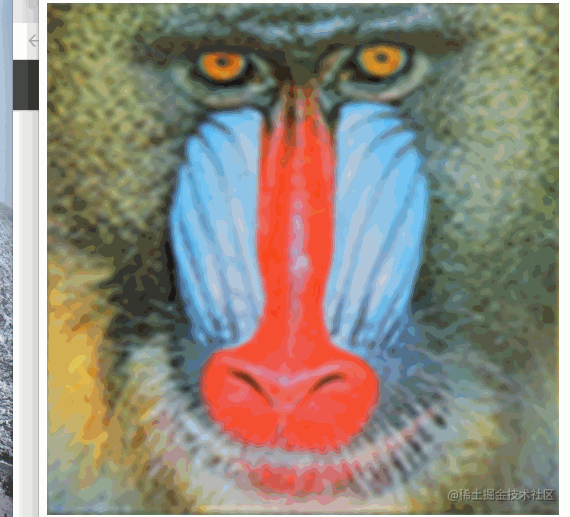# 快速高斯模糊算法的原理以及webgl工程实现FE @ 字节跳动

• 滤波和卷积(掩膜)
• 高斯模糊
• 快速高斯模糊原理

## 一、滤波和卷积

首先介绍一下滤波和卷积，滤波是信号处理中的一个概念，对于信号，可以由很多不同频率的波组成，滤波的本质就是增强或者减弱某一个频率的波，信号经过滤波处理，得到的新的信号。

简单来说滤波就是：从信号中得到指定波长的波

### (1)图像的时域和频域

那么什么是图片或者说图像中的滤波呢，首先我们要明白滤波其实是一个频域的概念，频域顾名思义就是频率，那么什么是图像的频率呢？

• 时域

要了解图像的频域首先先介绍一下图像的时域。

一幅数字图像可定义为一个二维函数f(x,y)，其中x和y是空间（平面）坐标，而在任何一对空间坐标（x,y）出的幅值f称为图像在该点处的灰度或强度。• 频域

图像的频率是表征图像中灰度变化剧烈程度的指标，是灰度在平面空间上的梯度。如：大面积的沙漠在图像中是一片灰度变化缓慢的区域，对应的频率值很低；而对于地表属性变换剧烈的边缘区域在图像中是一片灰度变化剧烈的区域，对应的频率值较高。简单来说：*频域就是灰度或者颜色变换的趋势(梯度) *。

同样我们以上面那幅图为例，我们用rgb颜色画出图片每一个点的颜色。可以得到如下图：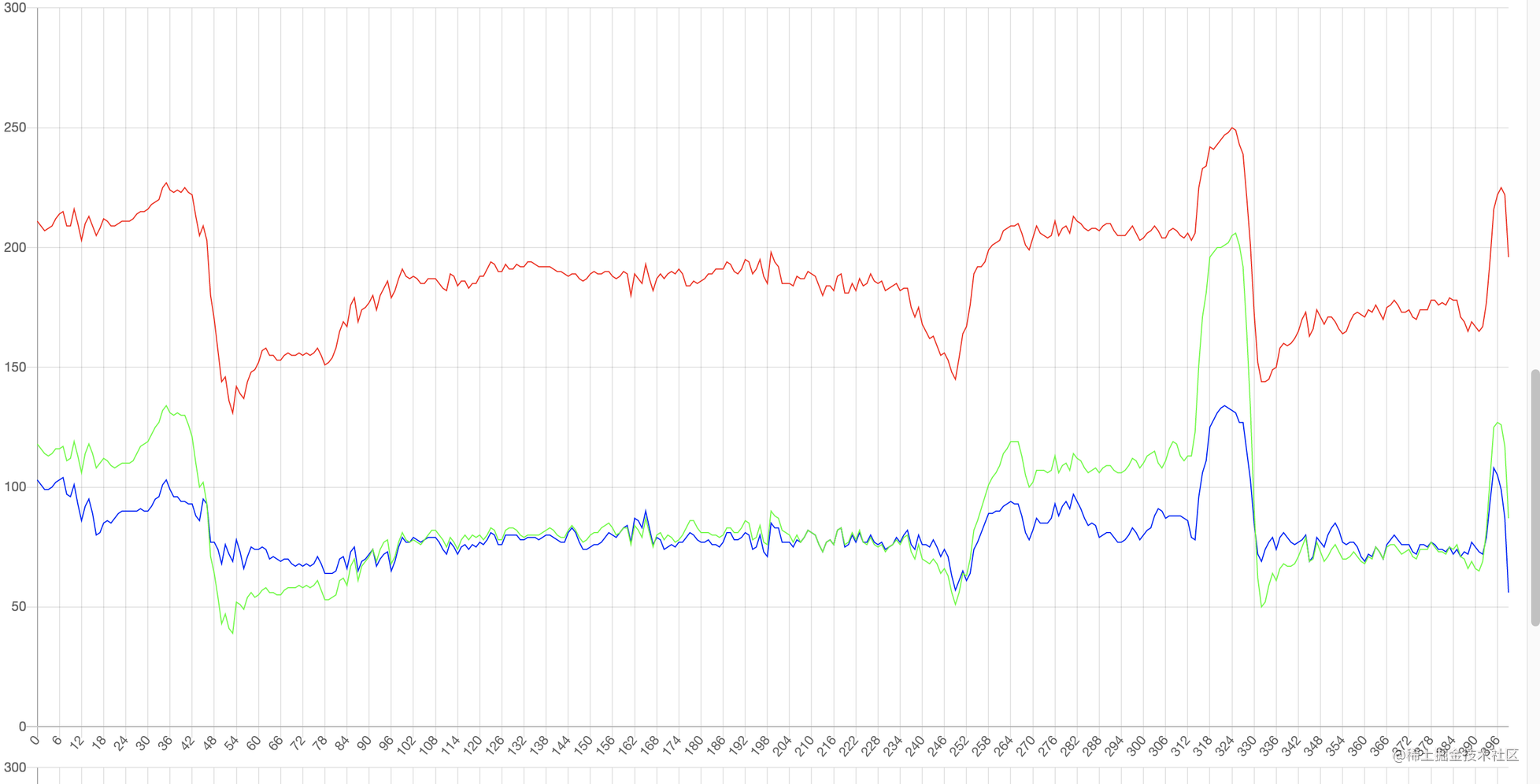颜色波动较大点就是频率较大的地方，相对的没有颜色波动的地方频率较小。

### (2)卷积(掩膜)

我们可以通过傅立叶变换将时域转换成频域，时域的卷积等于频的乘积。如果滤波可以在频域做，那么只需要做乘积。但是从时域到频域的转换并不是很直观，是相对复杂的，因此，在工程上对于图像的滤波大部分集中在时域，也就是对颜色值做卷积。

我们将原始图像，用一个窗口做卷积，得到的新的图像就是滤波后的图像，这个窗口就称为掩膜。这个窗口每个元素的值一般与滤波函数有关，我们接着举例来说明滤波的过程：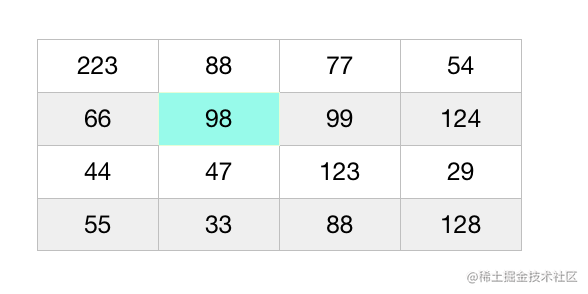上述就是一个简单的原始图像，图像上面的值表示灰度值，我们现在来假设一个掩膜。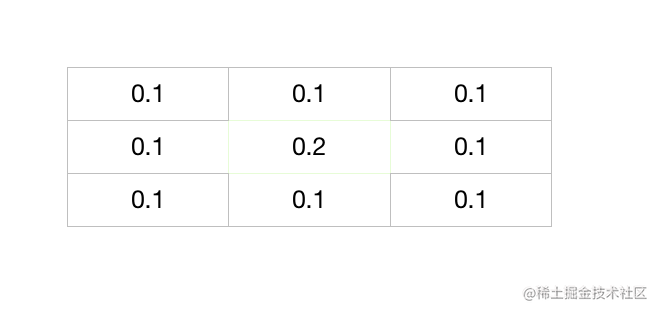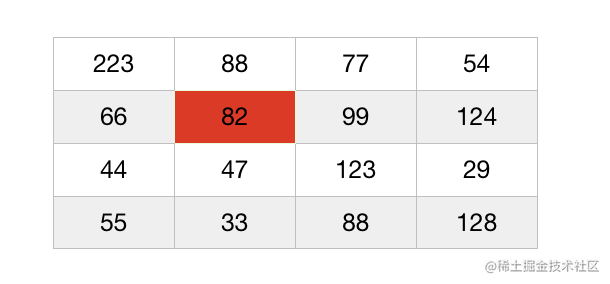## 二、高斯模糊

### (1)什么是高斯模糊

模糊就是一种特殊的滤波，经过这种滤波后图像变得不清晰。我们知道滤波 = 原始图像和掩膜的卷积，当掩膜(窗口)服从高斯分布时，此时我们称这种滤波为高斯滤波，也称为高斯模糊。

首先来看一维的高斯分布：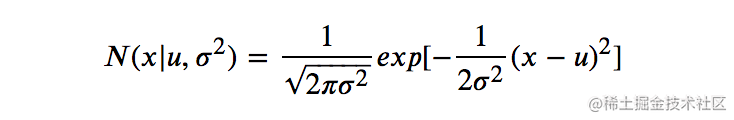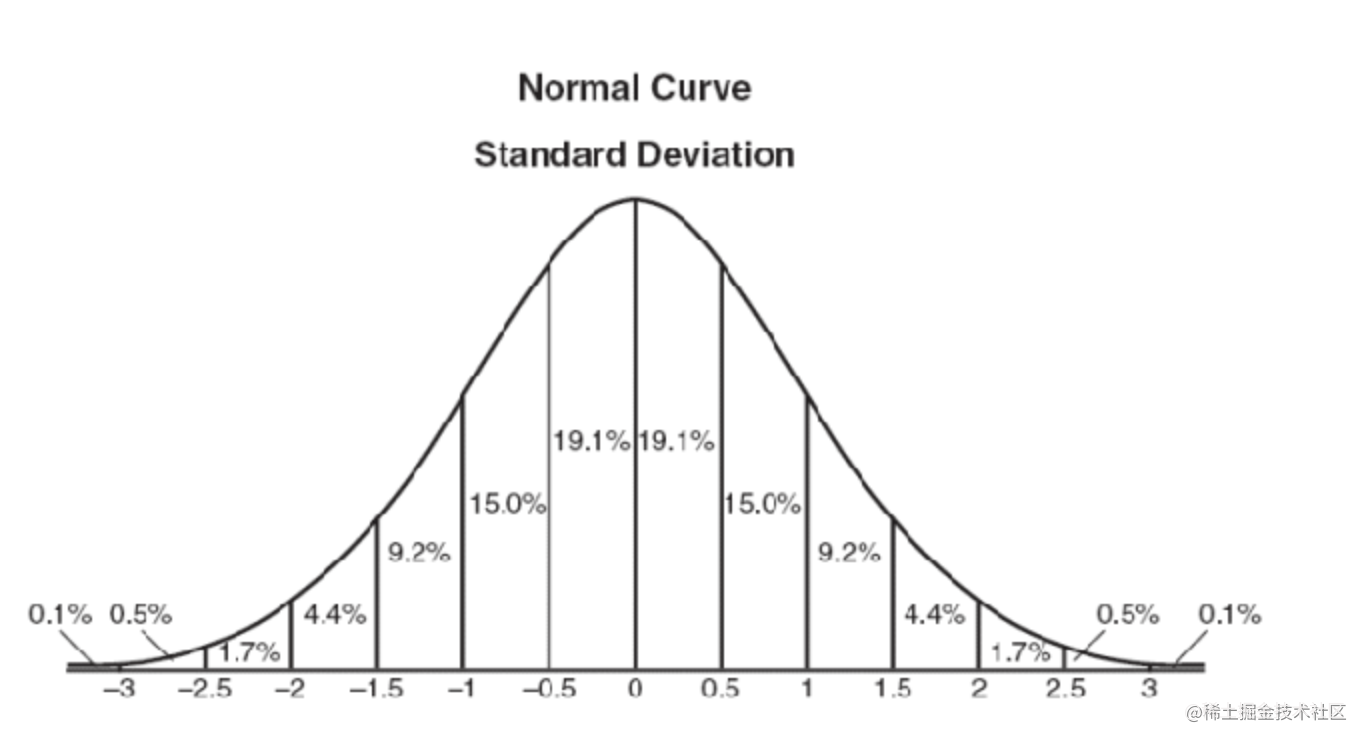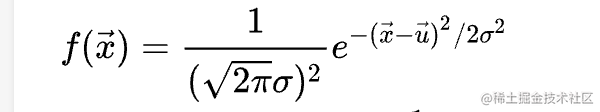• x 表示的是一个二维的坐标(x,y)。
• μ表示的是中心点的一般会假设称(0,0)。

在计算掩膜的时候，因为高斯模糊的本质是用周围的点来估计中心点，因此我们将需要被估计的那个中心点的坐标设置为(0,0)。其周边N个点的值，用高斯分布计算出来。就得到了滤波的窗口函数。我们以3x3滤波窗口的计算为例：我们取一个像素点为原点，然后采样其周边8个点，总共9个点。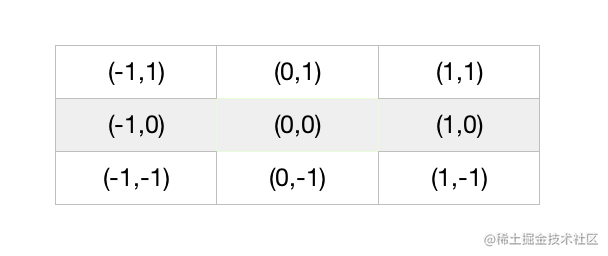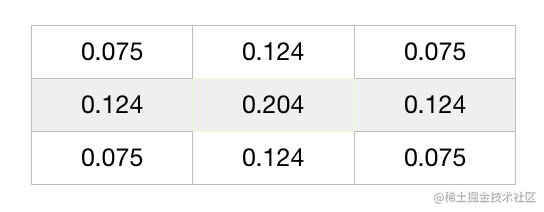### (2)高斯模糊的实现

39
525
749
981
11121
13169

``````      vec4 orColor=texture2D(u_image,v_texCoord);
const float PI = 3.1415926535897932384626433832795;
float w = 1./degree;
float temWeight;
temWeight = (1.0/(2.0 * PI * w * w));
temWeight = (1.0/(2.0 * PI * w * w)) * exp(-1 * w * w);
temWeight = (1.0/(2.0 * PI * w * w)) * exp(-2 * w * w);
float total;
total = temWeight + temWeight * 4. + temWeight * 4.
float orAlpha=orColor.a;
float weight;
weight = temWeight/total;
weight = temWeight/total;
weight = temWeight/total;
vec4 color= texture2D(u_image,v_texCoord + tex_offset * vec2( 0,0)) * weight
+ texture2D(u_image,v_texCoord + tex_offset * vec2( 0,-1)) * weight
+ texture2D(u_image,v_texCoord + tex_offset * vec2( 1,0)) * weight
+ exture2D(u_image,v_texCoord + tex_offset * vec2( -1,0)) * weight
+ texture2D(u_image,v_texCoord + tex_offset * vec2( 1, 0)) * weight
+ texture2D(u_image,v_texCoord + tex_offset * vec2( 1, 1)) * weight
+ texture2D(u_image,v_texCoord + tex_offset * vec2(-1, 1)) * weight
+ texture2D(u_image,v_texCoord + tex_offset * vec2( 1, -1)) * weight
+ texture2D(u_image,v_texCoord + tex_offset * vec2( -1, -1)) * weight

gl_FragColor=vec4(color.rgb,orAlpha);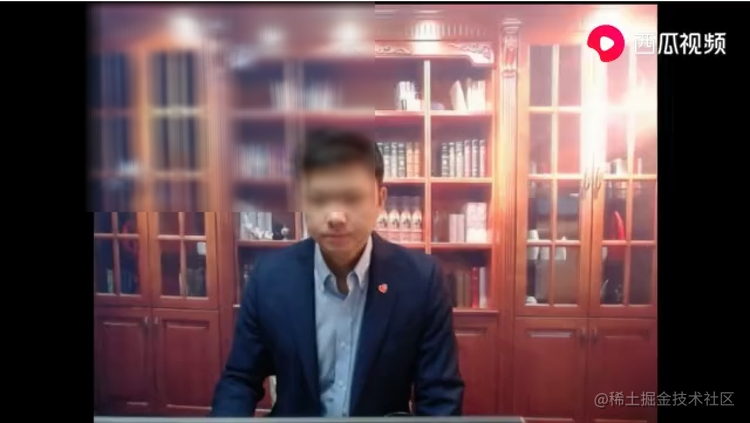## 三、快速高斯模糊原理

高斯模糊的计算量减化基本上围绕一下两个假设进行的：

1. 二维的高斯模糊可以看成两个一维高斯模糊的乘积
2. 标准差2σ的高斯模糊等于2个标准差为σ的高斯模糊之和

### （1） 杨辉三角(二项式系数)来代替权重求值

我们前面在计算权重的时候是用二维高斯分布来计算的，这个计算的过程可以简化成用二项式 系数来表示。原理就是：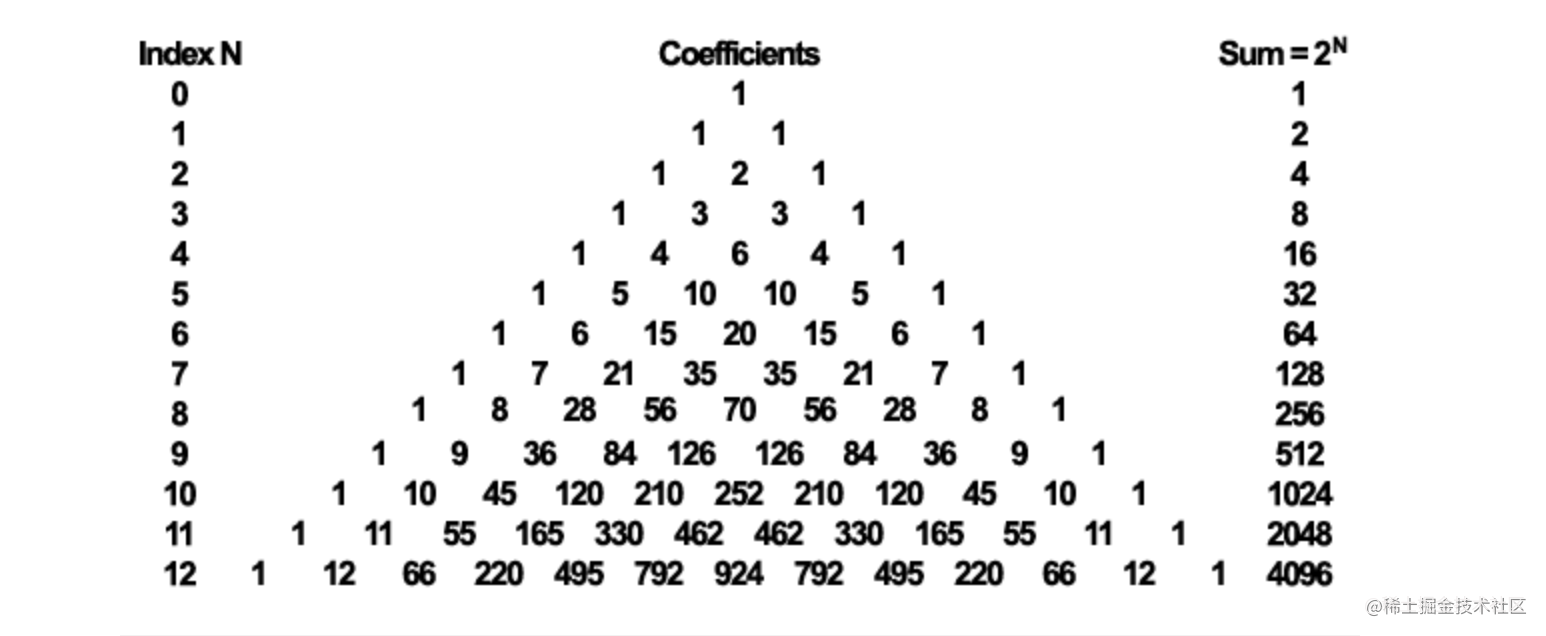### （2）二维高斯滤波拆成两个一维高斯滤波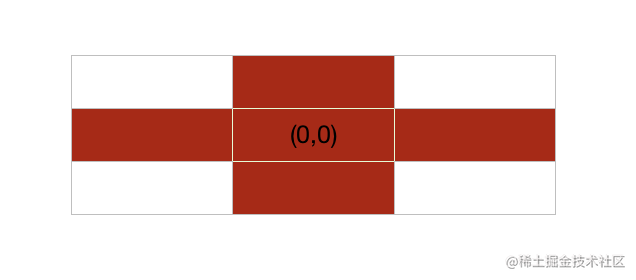### （3）线性滤波进一步减少计算

``````uniform sampler2D image;

out vec4 FragmentColor;

uniform float offset = float[](0.0, 1.0, 2.0, 3.0, 4.0);
uniform float weight = float[](0.2270270270, 0.1945945946, 0.1216216216,
0.0540540541, 0.0162162162);

void main(void) {
FragmentColor = texture2D(image, vec2(gl_FragCoord) / 1024.0) * weight;
for (int i=1; i<5; i++) {
FragmentColor +=
texture2D(image, (vec2(gl_FragCoord) + vec2(0.0, offset[i])) / 1024.0)
* weight[i];
FragmentColor +=
texture2D(image, (vec2(gl_FragCoord) - vec2(0.0, offset[i])) / 1024.0)
* weight[i];
}
}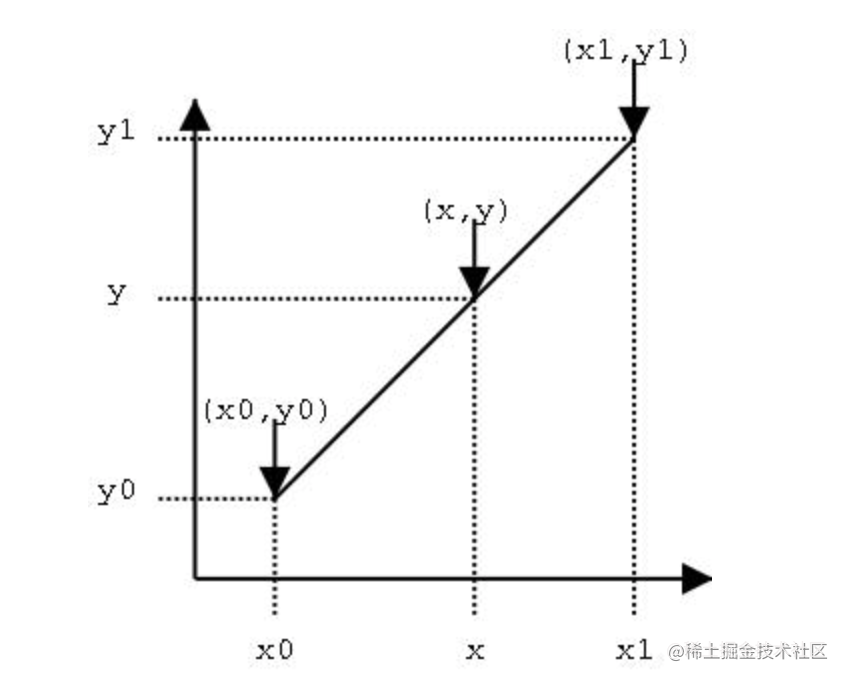y = y0 + [(y1-y0)/(x1-x0)] * x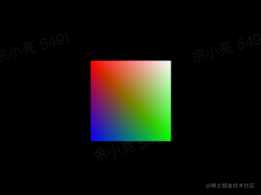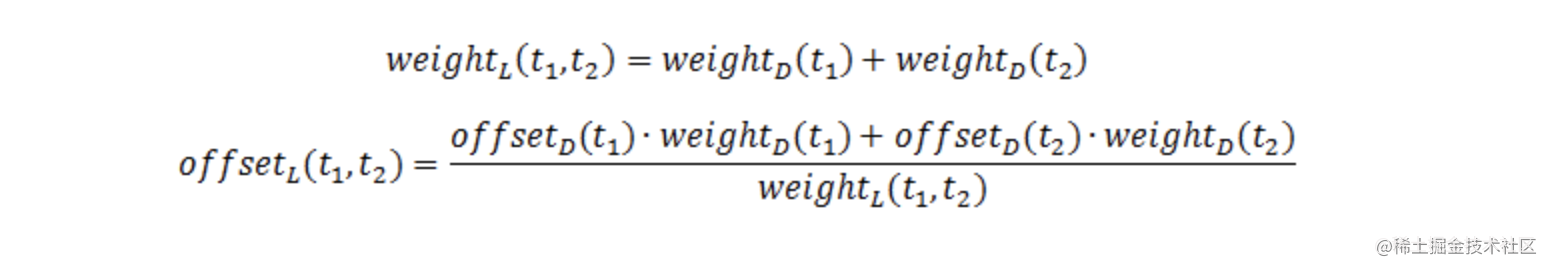``````uniform sampler2D image;

out vec4 FragmentColor;

uniform float offset = float[](0.0, 1.3846153846, 3.2307692308);
uniform float weight = float[](0.2270270270, 0.3162162162, 0.0702702703);

void main(void) {
FragmentColor = texture2D(image, vec2(gl_FragCoord) / 1024.0) * weight;
for (int i=1; i<3; i++) {
FragmentColor +=
texture2D(image, (vec2(gl_FragCoord) + vec2(0.0, offset[i])) / 1024.0)
* weight[i];
FragmentColor +=
texture2D(image, (vec2(gl_FragCoord) - vec2(0.0, offset[i])) / 1024.0)
* weight[i];
}
}

### （4）用空间换时间

• 对于半径为3的高斯模糊，窗口掩膜的系数是确定的。简化后只有2个不同的值。
• 对于像素而言，其像素值的范围也是确定的，0-255.

这样我们就可以 创建一个256 * 3的 map对象，保存在数组中，这样就不用去计算乘法，可以直接拿到掩膜后的值。这样就省去了5次乘法，进一步变成了只有4次加法。

### （5）最后FBO多进行几次